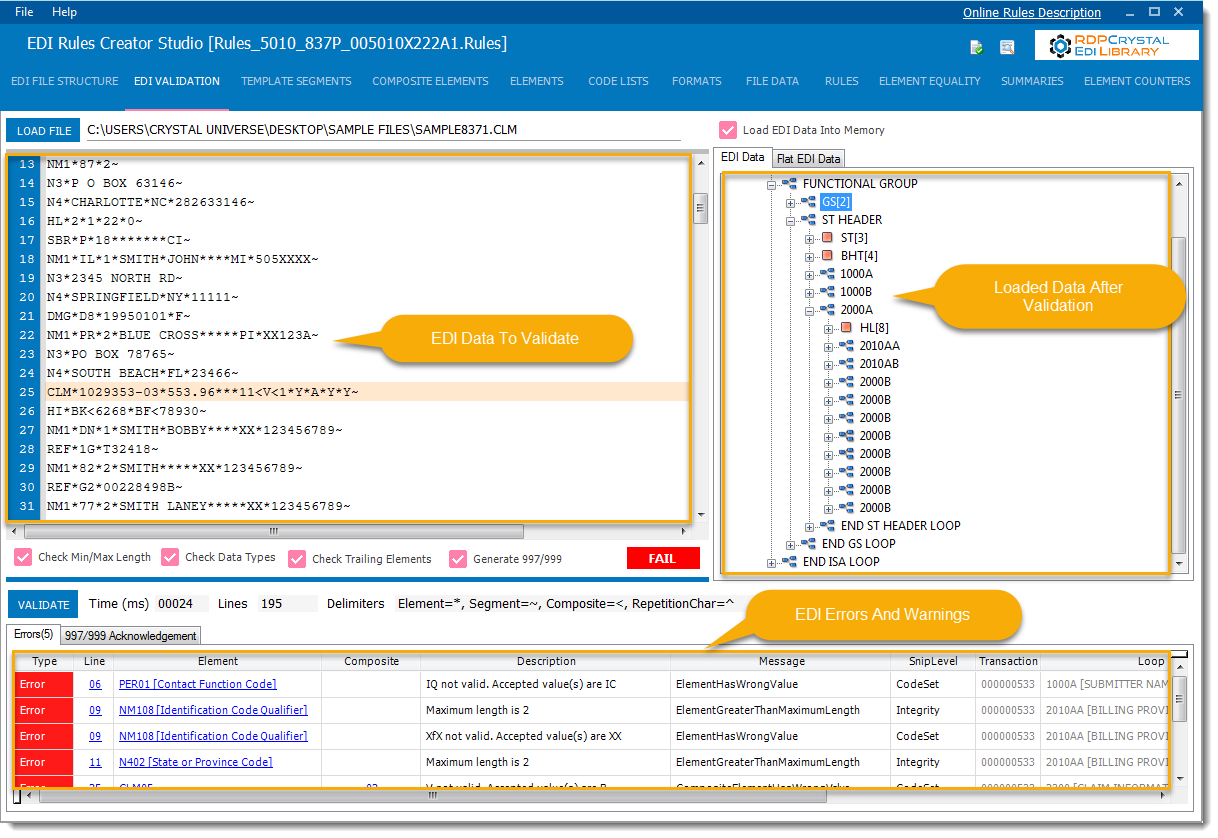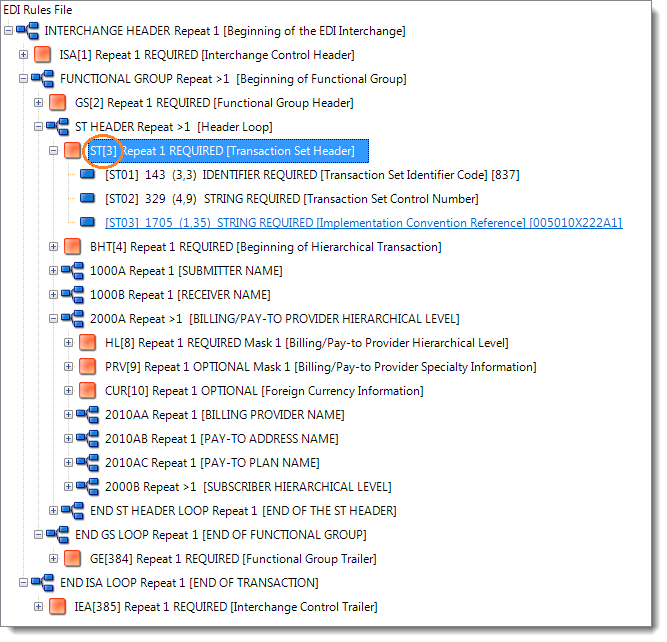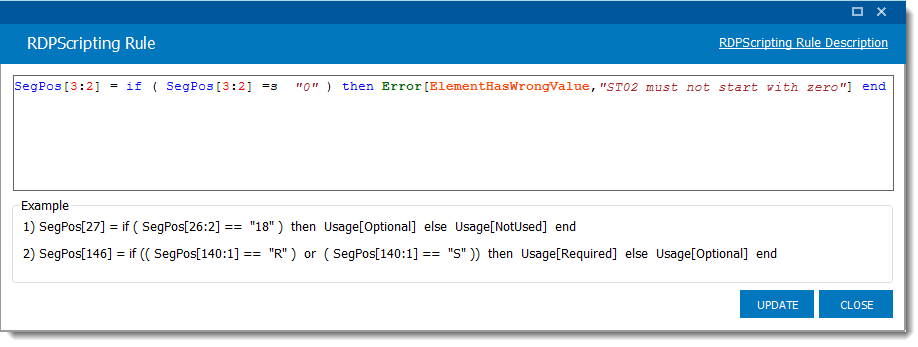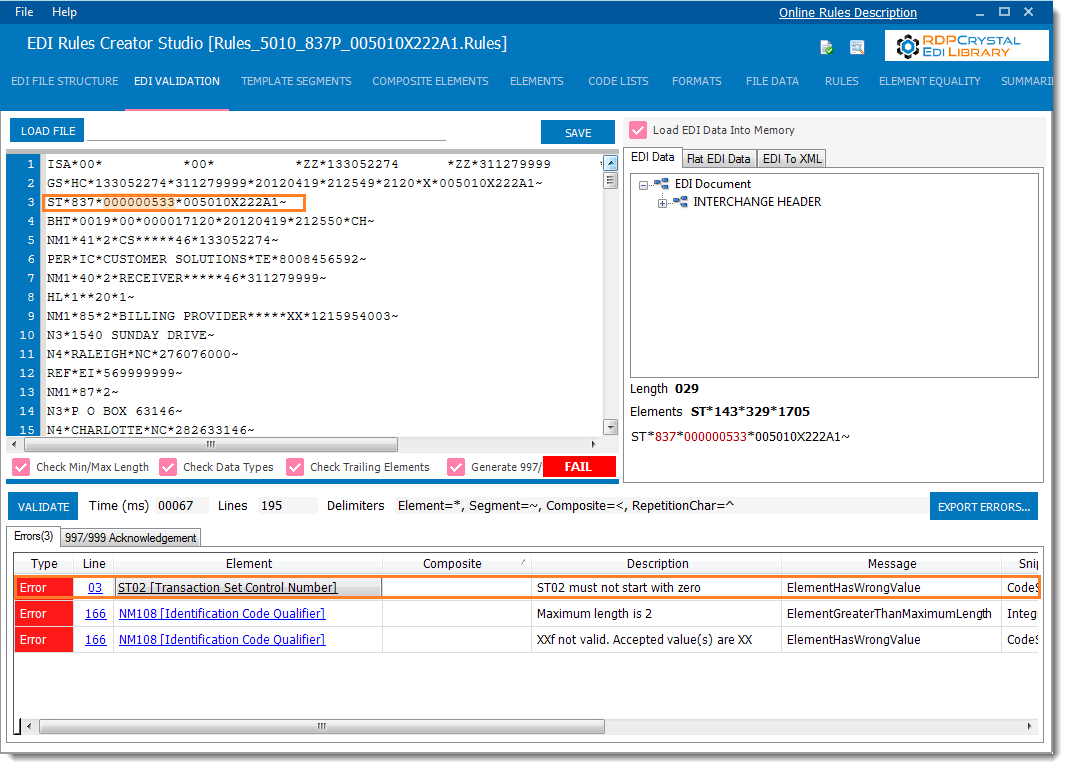RDPScript is the new powerful EDI scripting language that allows you to express your business logic around EDI validation more elegantly.  It allows you to add custom validation rules in simple, natural way.

In this tutorial we will demonstrate how to use RDPScript to add validation rules to an 5010 837 Professional EDI file.  We will use EDI Rules Creator Studio to create the RDPScript rules.##### EDI data we want to add custom rules for

ISA*00* *00* *ZZ*133052274 *ZZ*311279999 *120419*2125*^*00501*000002120*0*P*<~ GS*HC*133052274*311279999*20120419*212549*2120*X*005010X222A1~ ST*837*000000533*005010X222A1~ BHT*0019*00*000017120*20120419*212550*CH~ NM1*41*2*CS*****46*133052274~ PER*IC*CUSTOMER SOLUTIONS*TE*8008456592~ NM1*40*2*RECEIVER*****46*311279999~ HL*1**20*1~ NM1*85*2*BILLING PROVIDER*****XX*1215954003~ N3*1540 SUNDAY DRIVE~ N4*RALEIGH*NC*276076000~ REF*EI*569999999~ NM1*87*2~ N3*P O BOX 63146~ N4*CHARLOTTE*NC*282633146~

#### RDPScript Custom Rule: Check Element Value

In the above EDI data  we want to make sure that ST02, currently 000000553, does not start with a zero.  If we open an 5010 837 Rules file in EDI Rules Creator Studio we see that the  ST segment has an Segment Ordinal of 3.  The Segment Ordinal is how RDPScript uniquely identifies segments.The RDPScript rule we want to add looks like this

SegPos[3:2] = if ( SegPos[3:2] =s “0” ) then Error[ElementHasWrongValue,”ST02 must not start with zero”] end

The =s operator means ‘startsWith’.

See below to find out what the syntax means.  SegPos[3:2] means segment 3 element 2.

Let’s add our rule using EDI Rules Creator Studio’s Rules TabLets validate the data again by hitting the Validate buttonWe can clearly see that a validation error is now generated if ST02 starts with a 0.

RDPScript follows a well defined pattern and boils down to a if-then-end statement

### Syntax

if-then-end

`SegPos = if (Condition|CodeCondition) then Usage|LocalCode|Error end`
if-then-else-end

`SegPos = if (Condition|CodeCondition) then Usage|LocalCode|Error else Usage|LocalCode|Error end`

if-then-elseif-then-end

`SegPos = if (Condition|CodeCondition) then Usage|LocalCode|Error elseif (Condition) then Usage|LocalCode|Error end`

### Definitions

#### SegPos

The segment position we want to modifyOrdinal values are used.  For example SegPos is the ISA segment.  SegPos[1:2] will be the second element of segment ISASegPos[1:2:1] would be ISA02 composite element 1

#### Condition

A condition that must evaluate to true before the statement in the then clause can execute.

``+SegPos = if (SegPos[26:2] == "18") then Usage[Optional] else Usage[NotUsed] end``

In this scenario we want to change the usage of Segment Position 27 to Optional if Segment 26 Element 2 is equal to 18 otherwise mark it as Not Used

#### Complex Conditions

Two or more Conditions can be combined to form Complex Conditions using the or/and boolean operators

``+SegPos = if ((SegPos[140:1] == "R") or (SegPos[140:1] == "S")) then Usage[Required] else Usage[Optional] end``

Boolean Operations

 or Boolean OR and Boolean AND

#### Usage

A keyword used to modify the usage of a Segment PositionThe following usage values can be used

 Required Required Optional Optional NotUsed Not Used
##### Examples
``+SegPos = if (SegPos[26:2] == "18") then Usage[Optional] else Usage[NotUsed] end``
``+SegPos = if (SegPos[26:2] == "18") then Usage[Required] else Usage[Optional] end``
``+SegPos = if (SegPos[26:2] == "18") then Usage[Required] else Usage[NotUsed] end``

``+SegPos = if ((SegPos[140:1] == "R") or (SegPos[140:1] == "S")) then Usage[Required] else Usage[Optional] end``

#### LocalCode

A keyword used to modify the accepted values of elements

``+SegPos[3:1] = if ( SegPos[3:1] ==  "837" ) then LocalCode["we","w9"] end ``
``+SegPos[3:1] = if ( SegPos[3:1] ==  "837" ) then LocalCode["we","w9"] else LocalCode["837"] end``

In this scenario we are changing the accepted values of SegPos[3:1] to ‘we’ and ‘w9’ if it’s value is ‘837’

#### Error

A keyword used to create custom errors.  EDIValidator will generate an error if the condition evaluates to true

``+SegPos[135:5] = if (SegPos[135:5] == SegPos[40:5:1]) then Error[ElementHasWrongValue,"SV105 must be different from 2300 CLM05-01"] end``
``+SegPos[104:9] = if ((SegPos[26:9] != "") and (SegPos[104:9] != "")) then Error[ElementHasWrongValue,"SBR09 is used It should not be used after National PlanID is mandated"] end``
``+SegPos[275:2] = if ( SegPos[275:2] !=  SegPos[3:2] ) then Error[ElementHasWrongValue,"ST02 must be equal to SE02"] end elseif ( SegPos[275:2] !=  SegPos[3:3] ) then Error[ElementHasWrongValue,"ST02 must be equal to SE03"] end elseif ( SegPos[275:2] !=  SegPos[3:4] ) thenError[ElementHasWrongValue,"ST02 must be equal to SE04"] end else Error[ElementHasWrongValue,"ST02 must be equal to SE05"] end``

Custom error messages that can be used are listed below

`ElementHasWrongValue, ElementNotUsed, ElementRecommended, ElementNotRecommended, ElementMissing, CompositeElementHasWrongValue, CompositeElementNotUsed, CompositeElementRecommended, CompositeElementNotRecommended, CompositeElementMissing``SegmentNotUsed, SegmentMissing, SegmentRecommended, SegmentNotRecommended`

#### Checking for null

Check for existence of segments and elements

``+SegPos[135:5] = if (SegPos[135:5] == null) then Error[ElementRecommended,"This element is recommended"] end``
``+SegPos[135:5] = if (SegPos[135:5] != null) then Error[ElementNotUsed,"This element is not used"] end``

#### Length

The ‘l’ operator is used to check element lengths

```+SegPos[11:3] = if ( (SegPos[11:3] >l  "13") and (SegPos[11:3] then Error[ElementHasWrongValue,"length"]  end
```
In this scenario we are checking if the third element of segment 11 has a length greater than 13 but less than 16.  For a full list of length operations please see the Operators section below.

#### Contains

The ‘c’ operation is used to check if an element contains a certain character or text

`+SegPos[11:3] = if (SegPos[11:3] =c  "Provider") then Error[ElementHasWrongValue,"Cannot contain text Provider"]  end`
In this scenario we are checking if the third element of segment 11 contains the text ‘Provider’.  For a full list of string operations please see the Operators section below.

#### StartsWith

The ‘s’ operation is used to check if an element starts with a certain character or text

`+SegPos[11:3] = if (SegPos[11:3] =s  "P") then Error[ElementHasWrongValue,"Cannot start with P"]  end`
In this scenario we are checking if the third element of segment 11 contains the text ‘Provider’.  For a full list of string operations please see the Operators section below.

#### EndsWith

The ‘e’ operation is used to check if an element ends with a certain character or text

`+SegPos[11:3] = if (SegPos[11:3] =e  "xxx") then Error[ElementHasWrongValue,"Cannot end with xxx"]  end`
In this scenario we are checking if the third element of segment 11 contains the text ‘Provider’.  For a full list of string operations please see the Operators section below.

#### Operators

 == Equals != Not Equals > Greater Than < Less Than >= Greater Than Or Equal To <= Less Than Or Equal To =c Contains !c Does Not Contain =s Starts With !s Does Not Start With =e Ends With !e Does Not End With =l Length Equals !l Length Does Not Equals l Length Greater Than

#### Code Conditions – Custom Validating with .Net

.Net callbacks can be used for highly specialized validation scenarios using Code Conditions

``+SegPos[277:2] = if ( CodeCondition["shouldStartWithZeros"] ) then Error[ElementHasWrongValue,"Value should start with zeros"]  end``

Register for the appropriate event on the EDIValidator

``ediValidator.OnCodeCondition += new EDIValidator.CodeConditionEvent(ediValidator_CustomCondition);``

Write a custom validation check using .Net

``private void ediValidator_CustomCondition(object sender, CodeConditionEventArgs e)``{``  if (e.CodeCondition.Id == "shouldStartWithZeros")``{``     if (e.CurrentSegment.Elements.DataValue.StartsWith("0000")){``      e.ConditionValid = true;``     }``  }``}``

#### When Are Rules Are Executed

A Trigger SegPos is the segment that a rule belongs to.  Rules are executed when the Trigger SegPos is encountered while validation occurs.

``+SegPos = if (SegPos[26:2] == "18") then Usage[Required] else Usage[Optional] end``

The above rule is executed when segment 31 is encountered.

``+SegPos[3:1] = if ( SegPos[3:1] ==  "837" ) then LocalCode["we","w9"] end ``

The above the rule is executed when segment 3 element 1 is encountered

## RDPScript Short Hand

### Syntax

`[Segment/Element Position]=(Condition):ActionType:(true USAGE):(default USAGE)`
`[Segment/Element Position]=(Condition):ActionType: [Accepted Values]:[Default Values]`

ActionType is either `USAGE` or `LOCALCODE`

#### Examples

`27=26:2'EQ'18!USAGE!0!1`
`31=26:2'EQ'18!USAGE!2!0`
`40=26:2'EQ'18!USAGE!2!1`
`146=140:1'EQ'R,S!USAGE!2!0`
`207=26:2'NE'18!USAGE!2!1`
`321=315:1'EQ'R,S!USAGE!2!0`

The following `USAGE` numbers can be used to change the usage of a segment or element

 0 Optional 1 Not Used 2 Required 3 Recommended 4 Not Recommended

The following conditions can be used

 EQ Equal NE Not Equal GT Greater Than GE Greater Than Or Equal To LT Less Than LE Less Than Or Equal To EXISTS Exists NEXIST Not Exists

The different parts of the rule are separated by an exclamation character (!)

#### Rule

`24=23:2'EQ'18!USAGE!0!1`

Condition = `23:2'EQ'18` (If segment 23 element 2’s value is EQUAL to 18)
Action type=`USAGE` (We are going to modify the usage of segment 24)
True Usage = 0 (segment 24 is optional)
Default Usage = 1 (segment 24 is not used)

Meaning:If segment 23 element 2’s value is EQUAL to 18 then segment 24 usage is OPTIONAL else segment 24 is NOT USED

#### Rule

`28=23:2'EQ'18!USAGE!2!0`

Condition = 28:2’EQ’18 (If segment 23 element 2’s value is EQUAL to 18)
Action type=`USAGE` (We are going to modify the usage of segment 28)
True Usage = 2 (segment 28 is required)
Default Usage = 0 (segment 28 is optional)

Meaning: If segment 23 element 2’s value is EQUAL to 18 then segment 28 usage is REQUIRED else segment 28 is OPTIONAL

#### Rule

`40=23:2'EQ'18!USAGE!2!1`

Meaning: If segment 23 element 2’s value is EQUAL to 18 then segment 40 usage is REQUIRED else segment 40 is NOT USED

#### Rule

`212=23:2'NE'18!USAGE!2!1`

Meaning: If segment 23 element 2’s value is NOT EQUAL to 18 then segment 212 usage is REQUIRED else segment 212 is NOT USED

The condition can have more than one values

`212=23:2'EQ'18,19!USAGE!2!1`

Here we can see that the condition states that if segment 23 element 2
is equal to 18 OR 19 then segment 212 is REQUIRED else it is NOT USED.

#### Rule

`149:2=23:2'NE'18!USAGE!2!1`

Meaning: If segment 23 element 2’s value is NOT EQUAL to 18 then segment 149 element 2 usage is REQUIRED else NOT USED

#### Rule

`97:2=23'EXISTS'!USAGE!2!1`

Meaning: If segment 23 EXISTS then segment 97 element 2 usage is REQUIRED else NOT USED

#### LOCAL CODE

Rule

`149:2=23:2'NE'18!LOCALCODE!2,3,4,5,6!7,8,9,10`

#### `Meaning: If segment 23 element 2’s value is NOT EQUALto 18 then segment 149 element 2 accepted value are 2,3,4,5,6 else thedefault accepted values are 7,8,9,10`

Now that was easy!

Take Charge Of EDI

## RDPCrystal EDI Library